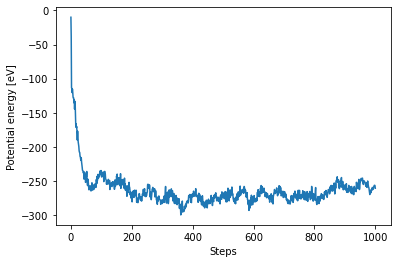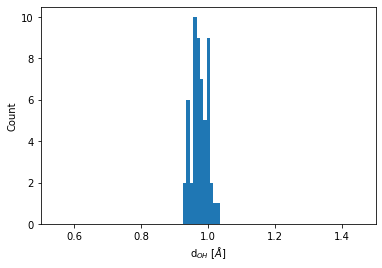# Molecular dynamics simulations of bulk water

In this example, we show how to perform molecular dynamics of bulk water using the popular interatomic TIP3P potential (W. L. Jorgensen et. al.) and LAMMPS.

:

import numpy as np
%matplotlib inline
import matplotlib.pylab as plt
from pyiron import Project
import ase.units as units
import pandas

:

pr = Project("tip3p_water")


## Creating the initial structure

We will setup a cubic simulation box consisting of 27 water molecules density density is 1 g/cm$$^3$$. The target density is achieved by determining the required size of the simulation cell and repeating it in all three spatial dimensions

:

density = 1.0e-24  # g/A^3
n_mols = 27
mol_mass_water = 18.015 # g/mol

# Determining the supercell size size
mass = mol_mass_water * n_mols / units.mol  # g
vol_h2o = mass / density # in A^3
a = vol_h2o ** (1./3.) # A

# Constructing the unitcell
n = int(round(n_mols ** (1. / 3.)))

dx = 0.7
r_O = [0, 0, 0]
r_H1 = [dx, dx, 0]
r_H2 = [-dx, dx, 0]
unit_cell = (a / n) * np.eye(3)
water = pr.create_atoms(elements=['H', 'H', 'O'],
positions=[r_H1, r_H2, r_O],
cell=unit_cell, pbc=True)
water.set_repeat([n, n, n])
water.plot3d()

:

water.get_chemical_formula()

:

'H54O27'


## Equilibrate water structure

The initial water structure is obviously a poor starting point and requires equilibration (Due to the highly artificial structure a MD simulation with a standard time step of 1fs shows poor convergence). Molecular dynamics using a time step that is two orders of magnitude smaller allows us to generate an equilibrated water structure. We use the NVT ensemble for this calculation:

:

water_potential = pandas.DataFrame({
'Name': ['H2O_tip3p'],
'Filename': [[]],
'Model': ["TIP3P"],
'Species': [['H', 'O']],
'Config': [['# @potential_species H_O ### species in potential\n', '# W.L. Jorgensen et.al., The Journal of Chemical Physics 79, 926 (1983); https://doi.org/10.1063/1.445869\n', '#\n', '\n', 'units real\n', 'dimension 3\n', 'atom_style full\n', '\n', '# create groups ###\n', 'group O type 2\n', 'group H type 1\n', '\n', '## set charges - beside manually ###\n', 'set group O charge -0.830\n', 'set group H charge 0.415\n', '\n', '### TIP3P Potential Parameters ###\n', 'pair_style lj/cut/coul/long 10.0\n', 'pair_coeff * * 0.0 0.0 \n', 'pair_coeff 2 2 0.102 3.188 \n', 'bond_style harmonic\n', 'bond_coeff 1 450 0.9572\n', 'angle_style harmonic\n', 'angle_coeff 1 55 104.52\n', 'kspace_style pppm 1.0e-5\n', '\n']]
})

:

job_name = "water_slow"
ham = pr.create_job("Lammps", job_name)
ham.structure = water
ham.potential = water_potential

/srv/conda/envs/notebook/lib/python3.7/site-packages/pyiron/lammps/base.py:170: UserWarning: WARNING: Non-'metal' units are not fully supported. Your calculation should run OK, but results may not be saved in pyiron units.
"WARNING: Non-'metal' units are not fully supported. Your calculation should run OK, but "

:

ham.calc_md(temperature=300,
n_ionic_steps=1e4,
time_step=0.01)
ham.run()

The job water_slow was saved and received the ID: 1

:

view = ham.animate_structure()
view


## Full equilibration

At the end of this simulation, we have obtained a structure that approximately resembles water. Now we increase the time step to get a reasonably equilibrated structure

:

# Get the final structure from the previous simulation
struct = ham.get_structure(iteration_step=-1)
job_name = "water_fast"
ham_eq = pr.create_job("Lammps", job_name)
ham_eq.structure = struct
ham_eq.potential = water_potential
ham_eq.calc_md(temperature=300,
n_ionic_steps=1e4,
n_print=10,
time_step=1)
ham_eq.run()

The job water_fast was saved and received the ID: 2

:

view = ham_eq.animate_structure()
view


We can now plot the trajectories

:

plt.plot(ham_eq["output/generic/energy_pot"])
plt.xlabel("Steps")
plt.ylabel("Potential energy [eV]");:

plt.plot(ham_eq["output/generic/temperature"])
plt.xlabel("Steps")
plt.ylabel("Temperature [K]");## Structure analysis

We will now use the get_neighbors() function to determine structural properties from the final structure of the simulation. We take advantage of the fact that the TIP3P water model is a rigid water model which means the nearest neighbors, i.e. the bound H atoms, of each O atom never change. Therefore they need to be indexed only once.

:

final_struct = ham_eq.get_structure(iteration_step=-1)

# Get the indices based on species
O_indices = final_struct.select_index("O")
H_indices = final_struct.select_index("H")

# Getting only the first two neighbors
neighbors = final_struct.get_neighbors(num_neighbors=2)


### Distribution of the O-H bond length

Every O atom has two H atoms as immediate neighbors. The distribution of this bond length is obtained by:

:

bins = np.linspace(0.5, 1.5, 100)
plt.hist(neighbors.distances[O_indices].flatten(), bins=bins)
plt.xlim(0.5, 1.5)
plt.xlabel(r"d$_{OH}$ [$\AA$]")
plt.ylabel("Count");### Distribution of the O-O bond lengths

We need to extend the analysis to go beyond nearest neighbors. We do this by using the number of neighbors in a specified cutoff distance

:

num_neighbors = final_struct.get_numbers_of_neighbors_in_sphere(cutoff_radius=9).max()

:

neighbors = final_struct.get_neighbors(num_neighbors)

:

neigh_indices = np.hstack(np.array(neighbors.indices)[O_indices])
neigh_distances = np.hstack(np.array(neighbors.distances)[O_indices])


One is often intended in an element specific pair correlation function. To obtain for example, the O-O coordination function, we do the following:

:

# Getting the neighboring Oxyhen indices
O_neigh_indices  = np.in1d(neigh_indices, O_indices)
O_neigh_distances = neigh_distances[O_neigh_indices]

:

bins = np.linspace(1, 8, 120)
count = plt.hist(O_neigh_distances, bins=bins)
plt.xlim(2, 4)
plt.title("O-O pair correlation")
plt.xlabel(r"d$_{OO}$ [$\AA$]")
plt.ylabel("Count");We now extent our analysis to include statistically independent snapshots along the trajectory. This allows to obtain the radial pair distribution function of O-O distances in the NVT ensamble.

:

traj=ham_eq["output/generic/positions"]
nsteps=len(traj)
stepincrement=int(nsteps/10)
# Start sampling snaphots after some equilibration time; do not double count last step.
snapshots=range(stepincrement,nsteps-stepincrement,stepincrement)

:

for i in snapshots:
struct.positions = traj[i]
neighbors = struct.get_neighbors(num_neighbors)
neigh_indices = np.hstack(np.array(neighbors.indices)[O_indices])
neigh_distances = np.hstack(np.array(neighbors.distances)[O_indices])
O_neigh_indices  = np.in1d(neigh_indices, O_indices)
#collect all distances in the same array
O_neigh_distances = np.concatenate((O_neigh_distances,neigh_distances[O_neigh_indices]))


To obtain a radial pair distribution function ($$g(r)$$), one has to normalize by the volume of the surfce increment of the sphere ($$4\pi r^2\Delta r$$) and by the number of species, samples, and the number density.

:

O_gr=np.histogram(O_neigh_distances,bins=bins)
dr=O_gr-O_gr
normfac=(n/a)**3*n**3*4*np.pi*dr*(len(snapshots)+1)
# (n/a)**3            number density
# n**3                number of species
# (len(snapshots)+1)  number of samples (we also use final_struct)

:

plt.bar(O_gr[0:-1],O_gr/(normfac*O_gr[0:-1]**2),dr)
plt.xlim(2, 7)
plt.title("O-O pair correlation")
plt.xlabel(r"d$_{OO}$ [$\AA$]")
plt.ylabel("$g_{OO}(r)$");[ ]: